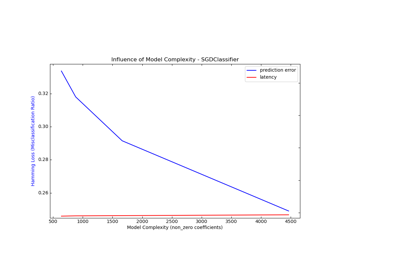# sklearn.metrics.hamming_loss¶

sklearn.metrics.hamming_loss(y_true, y_pred, *, sample_weight=None)[source]

Compute the average Hamming loss.

The Hamming loss is the fraction of labels that are incorrectly predicted.

Read more in the User Guide.

Parameters
y_true1d array-like, or label indicator array / sparse matrix

Ground truth (correct) labels.

y_pred1d array-like, or label indicator array / sparse matrix

Predicted labels, as returned by a classifier.

sample_weightarray-like of shape (n_samples,), default=None

Sample weights.

New in version 0.18.

Returns
lossfloat or int,

Return the average Hamming loss between element of y_true and y_pred.

Notes

In multiclass classification, the Hamming loss corresponds to the Hamming distance between y_true and y_pred which is equivalent to the subset zero_one_loss function, when normalize parameter is set to True.

In multilabel classification, the Hamming loss is different from the subset zero-one loss. The zero-one loss considers the entire set of labels for a given sample incorrect if it does not entirely match the true set of labels. Hamming loss is more forgiving in that it penalizes only the individual labels.

The Hamming loss is upperbounded by the subset zero-one loss, when normalize parameter is set to True. It is always between 0 and 1, lower being better.

References

1

Grigorios Tsoumakas, Ioannis Katakis. Multi-Label Classification: An Overview. International Journal of Data Warehousing & Mining, 3(3), 1-13, July-September 2007.

2

Wikipedia entry on the Hamming distance

Examples

>>> from sklearn.metrics import hamming_loss
>>> y_pred = [1, 2, 3, 4]
>>> y_true = [2, 2, 3, 4]
>>> hamming_loss(y_true, y_pred)
0.25


In the multilabel case with binary label indicators:

>>> import numpy as np
>>> hamming_loss(np.array([[0, 1], [1, 1]]), np.zeros((2, 2)))
0.75


## Examples using sklearn.metrics.hamming_loss¶